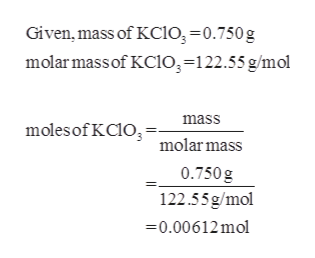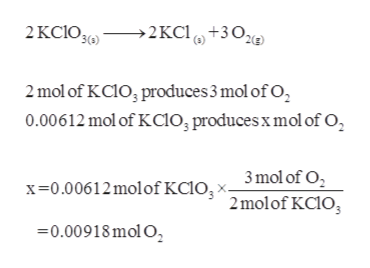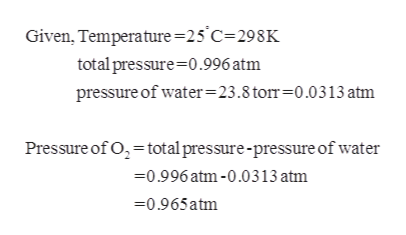# Small quantities of oxygen gas are generated in the laboratory by the following reaction in the presence of MnO2:2KCLO3(s)——> 2KCl(s)+3O2(g)What volume of oxygen is collected over water @ 25 degrees C by reaction of 0.750 g potassium chlorate if the corrected barometric pressure is 0.996atm? (Vapor pressure of water at 25 degrees C is 23.8 torr)

Question
16 views

Small quantities of oxygen gas are generated in the laboratory by the following reaction in the presence of MnO2:

2KCLO3(s)——> 2KCl(s)+3O2(g)

What volume of oxygen is collected over water @ 25 degrees C by reaction of 0.750 g potassium chlorate if the corrected barometric pressure is 0.996atm? (Vapor pressure of water at 25 degrees C is 23.8 torr)

check_circle

Step 1

The given mass of potassium chlorate can be converted into moles:help_outlineImage TranscriptioncloseGiven, mass of KC10, =0.750g molar massof KC1O,=122.55 g/mol mass molesof KC10, =. molar mass 0.750 g 122.55g/mol =0.00612 mol fullscreen
Step 2

The moles of oxygen produced can be calculated as follows:help_outlineImage Transcriptionclose2 KCIO3(,) →2KC1, +30e 2 mol of KC10, produces 3 mol of O, 0.00612 mol of Kcio, produces x mol of O, 3 mol of O, x=0.00612 molof KC1O, × 2 molof KC10, =0.00918 mol O, fullscreen
Step 3

The pressure of oxygen ca...help_outlineImage TranscriptioncloseGiven, Temperature=25°C=298K total pressure=0.996 atm pressure of water=23.8 torr=0.0313 atm Pressure of O, =total pressure-pressure of water =0.996 atm -0.0313 atm =0.965atm fullscreen

### Want to see the full answer?

See Solution

#### Want to see this answer and more?

Solutions are written by subject experts who are available 24/7. Questions are typically answered within 1 hour.*

See Solution
*Response times may vary by subject and question.
Tagged in

### Physical Chemistry